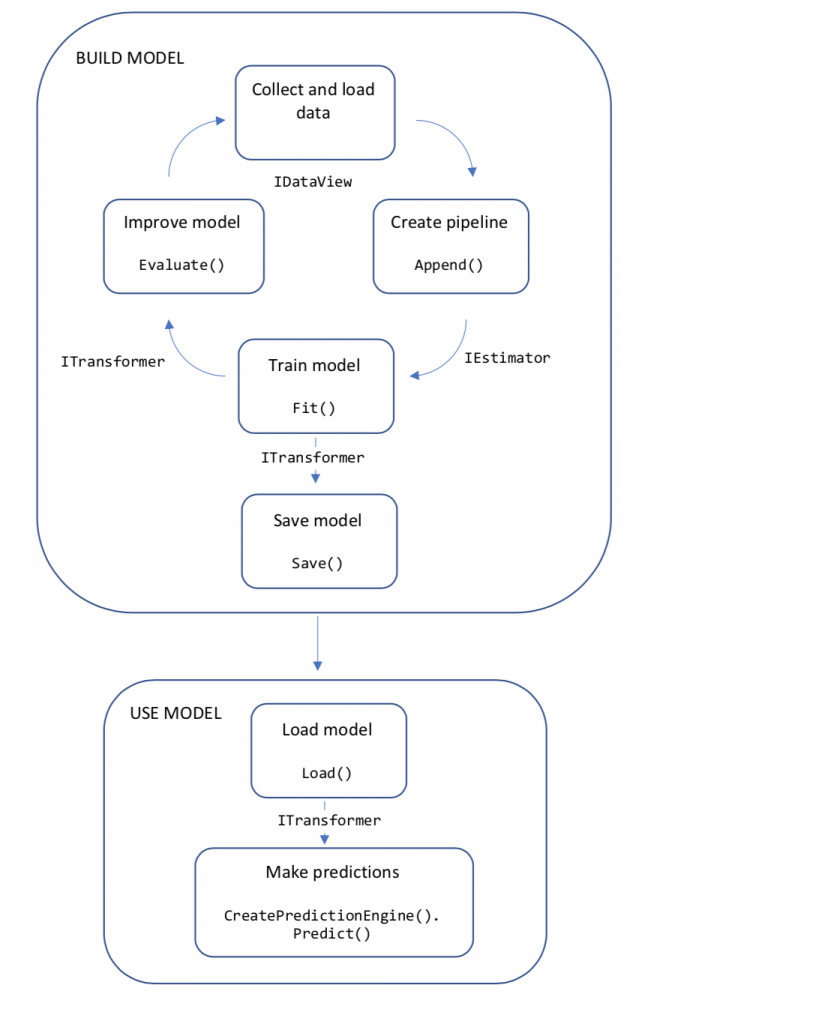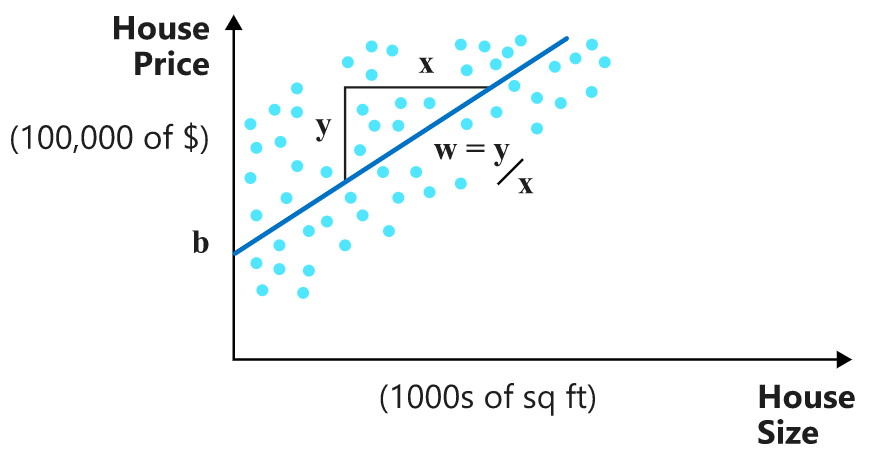﻿ ML.NET 机器学习 教程 线性回归模型 – 蒋智昊的博客# ML.NET 机器学习 教程 线性回归模型

using System;
using System.Collections.Generic;
using System.Linq;
using System.Text;
using Microsoft.ML;
using Microsoft.ML.Data;

namespace MLApp
{
internal class Program
{
public class HouseData
{
public float Size { get; set; }
public float Price { get; set; }
}

public class Prediction
{
[ColumnName("Score")]
public float Price { get; set; }
}

static void Main(string[] args)
{
MLContext mlContext = new MLContext();

// 1. Import or create training data
HouseData[] houseData = {
new HouseData() { Size = 1.1F, Price = 1.2F },
new HouseData() { Size = 1.9F, Price = 2.3F },
new HouseData() { Size = 2.8F, Price = 3.0F },
new HouseData() { Size = 3.4F, Price = 3.7F } };

// 2. Specify data preparation and model training pipeline
var pipeline = mlContext.Transforms.Concatenate("Features", new[] { "Size" }).Append(mlContext.Regression.Trainers.Sdca(labelColumnName: "Price", maximumNumberOfIterations: 100));

// 3. Train model
var model = pipeline.Fit(trainingData);

// 4. Make a prediction
var size = new HouseData() { Size = 2.5F };
var price = mlContext.Model.CreatePredictionEngine<HouseData, Prediction>(model).Predict(size);
// $作用是将{}内容当做表达式。 C 货币。 Console.WriteLine($"Predicted price for size: {size.Size * 1000} sq ft= {price.Price * 100:C}k");

// Predicted price for size: 2500 sq ft= \$261.98k

// 等待用户按下任意键。避免窗口关闭。
}
}
}• 将训练数据收集并加载到 IDataView 对象中。
• 指定操作的管道，以提取特征并应用机器学习算法。
• 通过在管道上调用 Fit() 来训练模型。
• 评估模型并通过迭代进行改进。
• 将模型保存为二进制格式，以便在应用程序中使用。
• 将模型加载回 ITransformer 对象。
• 通过调用 CreatePredictionEngine.Predict() 进行预测。ML.NET 模型是一个对象，它包含为了获得预测输出而要对输入数据执行的转换。